Courses

# Rigid pavement as per IRC 58-2002 (Part - 2) Civil Engineering (CE) Notes | EduRev

## Civil Engineering (CE) : Rigid pavement as per IRC 58-2002 (Part - 2) Civil Engineering (CE) Notes | EduRev

The document Rigid pavement as per IRC 58-2002 (Part - 2) Civil Engineering (CE) Notes | EduRev is a part of Civil Engineering (CE) category.
All you need of Civil Engineering (CE) at this link: Civil Engineering (CE)

Relative stiffness of slab to sub-grade
A certain degree of resistance to slab deflection is offered by the sub-grade. The sub-grade deformation is same as the slab deflection. Hence the slab deflection is direct measurement of the magnitude of the sub-grade pressure. This pressure deformation characteristics of rigid pavement lead Westergaard to the define the term radius of relative stiffness l in cm is given by the equation below.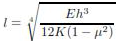where E is the modulus of elasticity of cement concrete in kg/cm2 (3.0×105), μ is the Poisson‟s ratio of concrete (0.15), h is the slab thickness in cm and K is the modulus of subgrade reaction.

Since the pavement slab has finite length and width, either the character or the intensity of maximum stress induced by the application of a given traffic load is dependent on the location of the load on the pavement surface. There are three typical locations namely the interior, edge and corner, where differing conditions of slab continuity exist. These locations are termed as critical load positions Equivalent radius of resisting section When the interior point is loaded, only a small area of the pavement is resisting the bending moment of the plate. Westergaard‟s gives a relation for equivalent radius of the resisting section in cm in the equation below.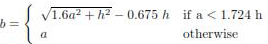where a is the radius of the wheel load distribution in cm and h is the slab thickness in cm Wheel load stresses - Westergaard‟s stress equation The cement concrete slab is assumed to be homogeneous and to have uniform elastic properties with vertical sub-grade reaction being proportional to the deflection. Westergaard developed relationships for the stress at interior, edge and corner regions, denoted as σi , σe , σc in kg/cm2 respectively and given by the equations below.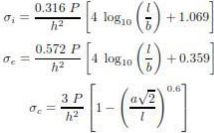where h is the slab thickness in cm, P is the wheel load in kg, a is the radius of the wheel load distribution in cm, l the radius of the relative stiffness in cm and b is the radius of the resisting section in cm.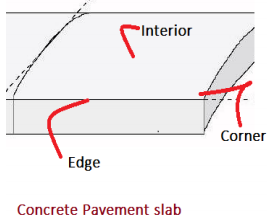Critcal stress locations

Temperature stresses
Temperature stresses are developed in cement concrete pavement due to variation in slab temperature. This is caused by (i) daily variation resulting in a temperature gradient across the thickness of the slab and (ii) seasonal variation resulting in overall change in the slab temperature. The former results in warping stresses and the later in frictional stresses.
Warping Stress
The warping stress at the interior, edge and corner regions, denoted as σti , σte , σtc in kg/cm2 respectively and given by the equations.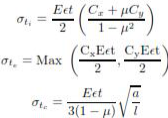where E is the modulus of elasticity of concrete in kg/cm2 (3×105), is the thermal coefficient of concrete per o C (1×107) t is the temperature difference between the top and bottom of the slab, Cx and Cy are the coefficient based on Lx/l in the desired direction and Ly /l right angle to the desired direction, μ is the Poisson‟s ration (0.15), a is the radius of the contact area and l is the radius of the relative stiffness.

Warping Stress are developed in C C pavement at different location :
During the day time when the slab is warped with convex upwards (Fig.l), the foundation pulls the slab down by spring action, the characteristic of the Winkler foundation. The stresses caused by the hypothetical tension in the spring are assumed to represent the curling or warping stress caused by self-weight of the slab. If the foundation is stiffer, the curling stress is large because the restraining force of the hypothetical spring foundation is also large though weight of the slab remains the same. The greater the temperature difference between the top and the bottom of the slab, greater is the warping and a large tension in the spring causes higher stresses. During the night hours, the slab curls as shown in Fig. 2. This condition is critical during the early life of concrete when it is very weak. Curling stresses are sensitive to the stiffness of the idealised foundation. Author‟s analysis shows that a concrete a slab laid on a Dry Lean Concrete (DLC) sub-base warps up and leave the foundation even for a temperature difference of 10°C between the top and the bottom of the slab and the self weight of the slab is not large enough to deflect the slab to make it rest on the foundation. Any higher temperature difference causes additional warping but does not cause any additional curling stresses since the self-weight remains the same.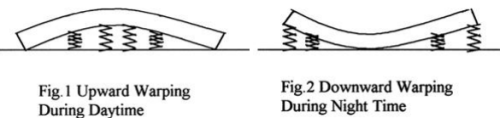Frictional stresses
The frictional stress σf in kg/cm2 is given by the equation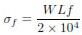where W is the unit weight of concrete in kg/cm2 (2400), f is the coefficient of sub grade friction (1.5) and Lis the length of the slab in meters.

Combination of stresses
The cumulative effect of the different stress give rise to the following thee critical cases
• Summer, mid-day: The critical stress is for edge region given by σcritical = σe + σte − σf
• Winter, mid-day: The critical combination of stress is for the edge region given by σcritical = σe + σte + σf
• Mid-nights: The critical combination of stress is for the corner region given by σcritical = σc + σtc

Offer running on EduRev: Apply code STAYHOME200 to get INR 200 off on our premium plan EduRev Infinity!

,

,

,

,

,

,

,

,

,

,

,

,

,

,

,

,

,

,

,

,

,

;